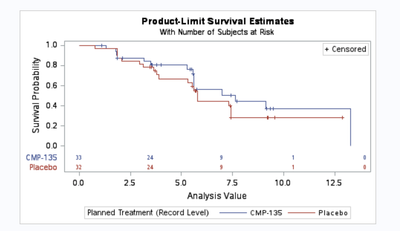## How to change interval for x-axis in the KM plot

Hi there,

I have been struggling with this problem for a while.

I run the PROC LIFETEST procedure below to get the KM plot. See the plot in the attachement. However, you will find that the time points along the x-axis are 0, 2.5, 5, 7.5, 9, and 12.5. But I want the time interval to be 3, which can made the time points to be 0,3, 6, 9, 12 and 15 so that the time points along x-axis is same as the time points for the number of at risk listing...I tried many options, even trying to revise PROC TEMPLATE. But none of them works......Does any one have resolved similar problem? Thanks in advance.

```ods output Survivalplot = SurvivalPlotData;
ods graphics on;
proc lifetest data=adtte1 plots=survival(atrisk=0 to 15 by 3);
time aval*cnsr(1);
run;
ods graphics off;```1 ACCEPTED SOLUTION

Accepted Solutionsdata_null__

## Re: How to change interval for x-axis in the KM plot

ATRISK <(options)> <=number-list> displays the numbers of subjects at risk at the given times. You can specify the following options: ATRISKTICK ATRISKLABEL guarantees that tick values are shown on the time axis for those times when the numbers of subjects at risk are displayed. If this option is not specified, you might not be able to tell at exactly which times the number of subjects at risk are displayed. If the ATRISKTICKONLY option is also specified, it takes precedence over the ATRISKTICK option. ATRISKTICKONLY specifies that tick values on the time axis be shown only at the times that are given in the ATRISK= list. If the ATRISKTICK option is also specified, it is ignored; that is, ATRISKTICKONLY takes precedence over ATRISKTICK.

2 REPLIES 2data_null__